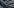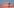# Performance of semi-structured data types in Snowflake

Snowflake extends the idea of traditional relational database by having the possibility to store and handle semi-structured data types. As shown in this post, semi-structured data types have effects on query performance and results of numerical operations.

On top of the traditional relational data, Snowflake also has support for semi-structured data with flexible schema. This can be utilized with for example following data formats: JSON and XML. Inside Snowflake, these are stored as either variant, array or object data types. Let us take a closer look what these mean.

Variant is a tagged universal type that can hold up to 16 MB of any data type supported by Snowflake. Variants are stored as columns in relational tables. Array is a list-like indexed data type that consists of variant values. Object, on the other hand, is a data type that consist of key-value pairs, where key is a not-null string and value is variant type data.

Snowflake provides guidelines on handling semi-structured data on their documentation. As a baseline, they recommend to store semi-structured data as variant data type, if usage for data is unsure. However, it is stated that when the usage is known, recommendation is to perform test cases to find solution with the best performance. Let us take a look what kind of differences might occur.

## Test setup

For testing purposes, we create three different tables:

1.   Table with 500 million rows
2.   Table with variant column having 500 million values
3.   Table with array column having 500 million values

The traditional table includes user identifier, which is a random field between values 1 and 5 million and amount field, which is integer value between values 1 and 500 million. Variant and array tables are both grouped tables. These are created with the traditional table as their source. Schema has user identifier and variant or array typed value field, which has aggregated list of the values for certain user identity. The tables are created with following queries:

```CREATE TABLE T_500M (
user_id   INT,
amount    INT);

INSERT INTO T_500M
SELECT UNIFORM (1, 5000000, random())
, UNIFORM (1, 500000000, random())
FROM TABLE ( GENERATOR ( ROWCOUNT => 50000000 ));

CREATE TABLE T_500M_VARIANT AS
SELECT user_id,
, CAST(ARRAY_AGG(amount) AS VARIANT) AS variant_field
FROM T_500M
GROUP BY user_id;

CREATE TABLE T_500M_ARRAY AS
SELECT user_id
,  ARRAY_AGG(amount) AS array_field
FROM T_500M
GROUP BY user_id;```

## Evaluating performance

Storing values as variant or array might seem like a good idea, if we want to aggregate sums on amount field for every user identity. As a query result, we want to show user identifier, count number of occurrences for that user and aggregated sum for the amount field. We can achieve it for each table with following queries:

```SELECT user_id
, COUNT(*) AS value_count
, SUM(amount) AS sum
FROM T_500M
GROUP BY user_id;

SELECT user_id
, COUNT(flat_variant.value) AS value_count
, SUM(flat_variant.value::INTEGER) AS sum
FROM T_500M_VARIANT
, lateral flatten(INPUT => variant_field) flat_variant
GROUP BY user_id;

SELECT user_id
, COUNT(flat_array.value) AS value_count
, SUM(flat_array.value::INTEGER) AS sum
FROM T_500M_ARRAY
, lateral flatten(INPUT => array_field) flat_array
GROUP BY user_id;```

Select-clause takes 15.6 seconds for the traditional relational table, 22.1 seconds with variant table and 21.9 seconds with array table. The difference is significant with the queries being over 40 % slower for semi-structured tables.

Another thing to consider with semi-structured formats is that queries on semi-structured data will not use result cache. We can notice this by running the queries again. The traditional table query takes only 0.2 seconds thanks to Snowflake’s persisted query results, but the queries to other tables take the same circa 22 seconds as earlier to complete.

The tested difference in our query time between structured data table and table with semi-structured data type exists, but it is still acceptable in some cases, where loading semi-structured data is a lot easier to variant or array columns. However, it needs to be noted, as stated in Snowflake documentation, query performance for data types that are not native for JSON are even worse for tables using variant or array. Shown test included only native JSON data types, but including for example datetime as variant would make the difference even bigger.

## Explicit data type conversion

It is important to pay attention to the data types when accessing array or variant data. Consider the following example, where we query total sum of the amount-field with following select-statement on the variant table:

```SELECT COUNT (*) AS row_count
, SUM(flat_variant.value) AS sum_without_cast
, SUM(flat_variant.value)::INTEGER AS cast_after_sum
, SUM(flat_variant.value::INTEGER) AS cast_before_sum
FROM T_500M_VARIANT
, lateral flatten(INPUT => variant_field) flat_variant;```

For query results, we get three different sums:
No specified casting:           1.24998423949572e+17
Casting before sum:            124998423949572384
Casting after sum:               124998423949572368

The same sum amounts are received when running the above query for the array table. Difference comes from Snowflake’s calculations, where variant and array are handled using JavaScript. JavaScript language uses Float as data type for numeric values. As shown in the earlier blog post using floating-point numeric data types, may lead to imprecise values. Even though the table only has fixed-point numeric values, using variant or array converts them to floating points unless determined explicitly when querying data.

## Conclusion

Possibility to store semi-structured data in relational tables on Snowflake comes in handy for many business needs that do not have traditional relational source data. It enables loading semi-structured data straight to Snowflake and parsing data onwards from there. Even though this is possible, it should be tested per use case whether it is the best solution.

When evaluating query performance we noticed that querying tables with semi-structured data types, the select-clauses resulted in being 40 % slower compared to similar table with structured data types. This is with JavaScript native data types, while non-native types will result in even bigger difference for execution time. Semi-structured data types can’t  utilize result cache, so re-running the queries will take similar time as the initial one.

Best practice is converting data types explicitly, when accessing variant or arrays. Snowflake engine uses JavaScript to handle these data types, so as was shown, numeric values may suffer from rounding.# To float, or not to float – Choosing correct numeric data type in Snowflake

Fixed-point numerical data types should be the default choice when designing Snowflake relational tables. Using floating-point data types has multiple downsides, which are important to understand. The effect of choosing different numerical data type can be easily tested.

Snowflake numeric data types can be split in two main categories: fixed-point numbers and floating-point numbers. In this blog post we are going to look at what these mean from Snowflake database design point of view, and especially should you use floating type when dealing with numeric data?

Fixed-point numbers are exact numeric values, which include data types such as number, integer and decimal. For these data types, developer can determine precision (allowed number of digits in column) and scale (number of digits right of the decimal point). In Snowflake, all fixed-point numeric data types are actually type decimal with precision 38 and scale 0, if not specified differently. Typical use cases for fixed-point data types are natural numbers and exact decimal values, such as monetary figures, where they need to be stored precisely.

On the other side of the spectrum are floating-point numbers, which are approximate representations of numeric values. In Snowflake, floating-point columns can be created with key-words float, double, double precision or real. However, as the Snowflake documentation states, all of these data types are actually stored as double and they do not have difference in precision, but displayed as floats. Floating-point data types are mainly used in mathematics and science to simplify the calculations with scientific notation. Storing numbers with major differences in magnitude is their advantage in databases too, because zeros trailing or following the decimal sign does not consume memory as it does for decimal format. In traditional computing, floats are considered faster for computation, but is that really the case in modern database design?

## Floating-point precision

First, let us explore inserting data on Snowflake into table with float as numeric data type. We create a table and insert 500 million rows of generated dummy data to the table with following query:

```CREATE TABLE T_FLOAT_500M (
id            INT,
float_field   FLOAT );

INSERT INTO T_FLOAT_500M
SELECT SEQ8()
, UNIFORM(1, 500000000, RANDOM())::FLOAT
FROM TABLE ( GENERATOR ( ROWCOUNT => 500000000 ) );```

To see the effect of using float as the data type for big numeric values, we can run the following query:

```SELECT SUM(float_field) AS NO_CONVERSION
, SUM(float_field::INT) AS CONVERT_VALUES_SEPARATELY
, SUM(float_field)::INT AS CONVERT_SUM_VALUE
FROM T_FLOAT_500M;```

Sum without the conversion produces us a rounded number with scientific notation: 1.24997318579082e+17
Separately converted values sum produces result: 124997318579081654
Conversion made after the calculation produces sum value: 124997318579081664

From the sum results, we will notice the accuracy problem related to storing numeric values as floats as the sum results differ from each other. When dealing with large or extremely accurate numeric values, floats may cause differentiation in results due to their nature of being approximate representations. Same effect can be seen when using WHERE clauses as the approximate representations may not work as designed with conditions that point to exact numeric values.

## Storage size of float

Next, we create a similar table as earlier, but with the second field being type integer and populate it without converting random figures to floats.

```CREATE TABLE T_INT_500M (
id          INT,
int_field   INT);

INSERT INTO T_INT_500M
SELECT SEQ8()
, UNIFORM(1, 500000000, RANDOM())::INT
FROM TABLE ( GENERATOR ( ROWCOUNT => 500000000 ) );

SHOW TABLES LIKE '%_500M';```

Looking at the Snowflake table statistics, we will notice integer table is smaller (3.37 GB) compared to the float table (5.50 GB). The difference in table sizes is significant with the float table being 63 % bigger. This can be explained by Snowflake reserving 64 bits of memory for every float value. Integer values on the other hand are stored in compressed format and take only the necessary amount of memory.

This difference is seen also on SELECT queries, where querying all rows with X-Small warehouse takes only 85 seconds for integer type table compared to the 160 seconds with the float type table. Difference is once again major.

## Summary

Floats still have their use cases with numbers that have majorly different magnitudes. But from the presented test cases, we can draw a conclusion that using floating-point data types will lead to bigger storage sizes and longer query times, which result as an increase to data warehousing costs. Another thing to consider is the possibility of imprecise values, when dealing with extremely accurate data. Whenever possible, it is recommended not to use float as a type for numeric data without a specific reason. Especially precise and whole numbers are not meant to be stored as floats and should be given appropriate data types.